#### Theory of Bipolar Junction Diode II

Forward Bias Conditions:

Whenever an external electric field is applied to p-n junction, that is positive with respect to p-type and negative with respect to n-type, then the device is said to be forward biased as shown in figure below. In this case, the applied electric field tends to counteract the built-in field that lowers the potential barrier between n-type and p-type materials by an amount equivalent to the applied voltage. This lowers the barrier that opposes current flow and permits a raised number of electrons to cross the junction and diffuse into p-region. Similarly a raised number of holes can cross the junction and diffuse the n-region. This has the effect of increasing the minority carrier concentration in both the regions above equilibrium level. The raised levels of carrier concentration are maintained as long as the external bias voltage is applied. The carriers which re-join as they diffuse to the neutral regions of diode are substituted by an external supply to maintain the raised carrier concentrations. These outcomes in a continuous flow of current in the forward direction from p-type to n-type via diode.

Reverse Bias Conditions:

Whenever an external electric field is applied to p-n junction that is negative with respect to p-type and positive with respect to n-type, the device is state to be reverse biased (as shown in figure below). In this case, the applied field tends to improve the built-in field that raises the potential barrier between n-type and p-type materials by an amount equivalent to the applied voltage. In this situation, charge carriers are drawn further away from the junction that lowers their concentrations in this area from their unbiased equilibrium levels towards zero. Though, there is still a small amount of minority carriers produced in each area, due to thermal agitation, which can cross the junction and contribute to a small leakage current in reverse direction termed as the reverse saturation current.

Physical Model: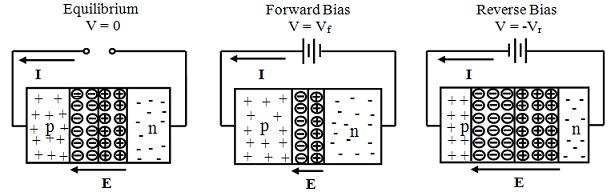Reverse Bias V = - Vr and V = -Vf

Barrier Potential: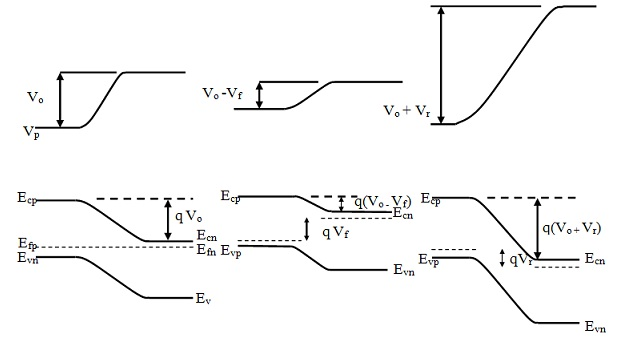Minority Carrier Concentration: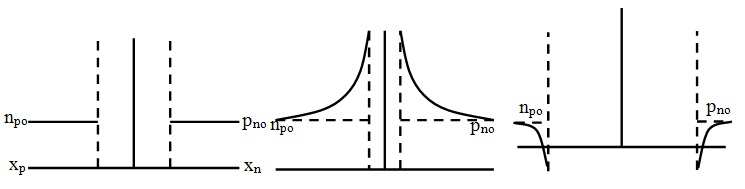Figure: Bias Conditions of a p-n Junction with Na ≈ Nd

Charge Transport under Biased Conditions:

The built-in electric field in p-n junction is very high, of the order of 1000 kV/m (1MV/m). Therefore, whenever an external electric field is applied to bias the device, then the applied field is developed almost wholly across the junction or depletion area and only a very small field present all along the main p-type and n-type regions of the diode as can be seen in figure below. This signifies that carriers crossing the junction do so by a drift method whereas carriers passing via the main neutral regions do so, primarily, by the diffusion method. This signifies that at boundaries on either side of the junction, the condition is efficiently one where surplus minority carriers are being injected to the non-ionized doped areas and start to diffuse into such regions, re-joining with majority carriers as they do so. Surplus holes diffuse into the n-type region whereas surplus electrons diffuse into p-region.

Carrier Injection beneath Bias Conditions:

As discussed, excess minority carriers injected via a boundary into a volume of semiconductor material rich in majority carriers diffuse the material, rejoining with majority carriers as they do so. Hence an exponential minority carrier concentration profile in the volume is set up in question. Figure below exhibits the condition for forward-biased p-n junction where there is an exponential distribution of surplus electrons into the p-region away from the junction and an identical distribution of surplus holes into the n-region away from the junction. Note that the exponential distribution applies to surplus carriers and, therefore, sits on top of unbiased, normal equilibrium levels.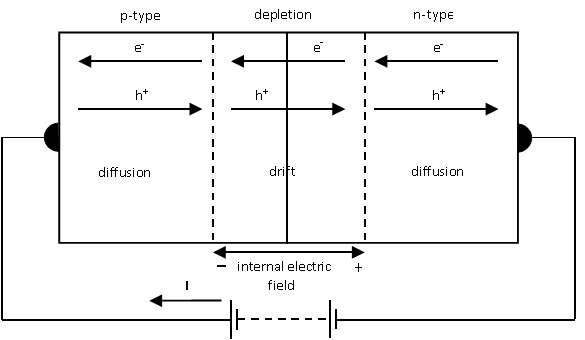Figure: Current Flow Mechanisms in Forward Biased p-n Diode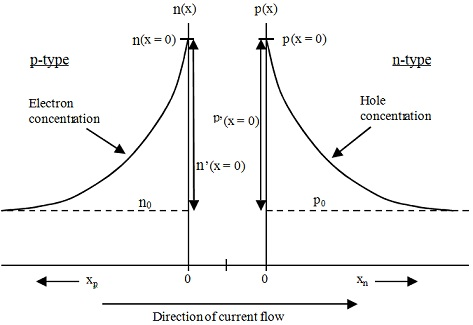Figure: Carrier Concentration Profiles in a Forward Biased p-n Diode

This can be shown that whenever a bias voltage V, is applied to the junction, the probability of a carrier crossing the junction is altered by a factor of eqV/kT = eV/VT. As a result, the concentration of minority carriers on either side of the junction is as well modified by similar factor from the unbiased, equilibrium value. The profiles of surplus carrier concentrations can, thus, be associated to the bias voltage V, applied to the p-n junction as:

A) Concentrations at boundaries:

n(x = 0) = n0eV/VT           ;    p(x = 0) = p0eV/VT

B) Surplus concentrations at boundaries:

n’(x = 0) = n(x = 0) - n0    ;    p’(x = 0) = p(x = 0) - p0

n’(x = 0) = n0(eV/VT - 1)    ;    p’(x = 0) = p0(eV/VT - 1)

C) Surplus concentration as functions of distance:

n’(x) = n0(eV/VT - 1) e-x/Ln    ;    p’(x) = p0(eV/VT – 1) e-x/Lp

The Ideal Diode Equation:

This is desired to derive a current-voltage relationship for the p-n diode and hence it can be characterized as an electrical circuit element. Then, an expression for total terminal current via the device should be obtained. When current flow in neutral regions of the device is taken to be by diffusion merely, then the total current due to applied bias can be taken as the sum of diffusion currents due to both carrier types, flowing at the boundaries of the junction with neutral regions (prior to recombination has taken place). This was shown formerly that the diffusion fluxes for holes and electrons are given as:

Jn diff = q Dn (dn/dx) and Jp diff = - q Dp (dp/dx)

This is the condition for holes and electrons traveling in similar x-direction. In case of forward biased diode, the holes and electrons are traveling in opposite directions. When the positive x-direction is taken as the direction of conventional current flow, that is, the direction of flow for positive charge, then the net terminal current due to surplus charge carrier injection is as follows:

I = - q A Dn [dn’(x)/dx] |x = 0  –q A Dp [dp’(x)/dx] |x = 0

Replacing for n’(x) and p’(x) gives: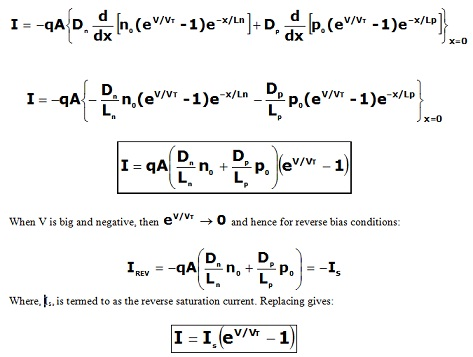This is termed as Ideal Diode Equation and characterizes the current-voltage relationship of the diode as electrical circuit element.

The Ideal Diode relationship is as shown plotted in figure below. From the curve it can be observe that whenever the diode is forward biased, the regular exponential relationship can eagerly be identified. The degree of current conduction beneath this condition depends basically on the doping concentrations employed in the semiconductor materials. Whenever the diode is reverse biased with the applied voltage V negative, the exponential factor is negligible and the current levels off at reverse saturation value –IS. This value is small and it can be observed that once a small value of reverse voltage is exceeding, the current becomes independent of voltage. This current is basically a leakage current present due to thermally produced carriers.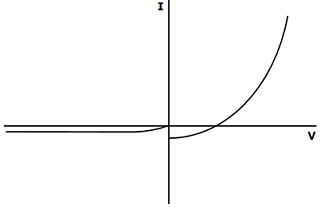Figure: Current-Voltage Relationship for the Ideal Diode

Latest technology based Electrical Engineering Online Tutoring Assistance

Tutors, at the www.tutorsglobe.com, take pledge to provide full satisfaction and assurance in Electrical Engineering help via online tutoring. Students are getting 100% satisfaction by online tutors across the globe. Here you can get homework help for Electrical Engineering, project ideas and tutorials. We provide email based Electrical Engineering help. You can join us to ask queries 24x7 with live, experienced and qualified online tutors specialized in Electrical Engineering. Through Online Tutoring, you would be able to complete your homework or assignments at your home. Tutors at the TutorsGlobe are committed to provide the best quality online tutoring assistance for Electrical Engineering Homework help and assignment help services. They use their experience, as they have solved thousands of the Electrical Engineering assignments, which may help you to solve your complex issues of Electrical Engineering. TutorsGlobe assure for the best quality compliance to your homework. Compromise with quality is not in our dictionary. If we feel that we are not able to provide the homework help as per the deadline or given instruction by the student, we refund the money of the student without any delay.

2015 ©TutorsGlobe All rights reserved. TutorsGlobe Rated 4.8/5 based on 34139 reviews.